### Ilana L. Abrahamson, Cara R. Nelson, and David L. R. Affleck. 2011. Assessing the performance of sampling designs for measuring the abundance of understory plants. Ecological Applications 21:452–464.

Appendix C. Proof of unbiasedness of the Strip Adaptive Cluster Sampling (SACS) Estimator.

Define the population of interest as the grid of G equal-sized cells and let yl denote the attribute value for the lth cell (l = 1, 2, …, G). An adaptive cluster sampling design divides this population into K mutually exclusive networks Nk (k = 1, 2, …, K) each comprised of sk cells (Sk sk = G) with attribute totals zk = S lÎNk yl . For the derivations below it is useful to evenly distribute the network totals among component cells by defining the variable as the mean amount of attribute (zk /sk) for the network to which cell l belongs. The population mean is: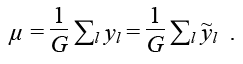With SACS, the primary sampling unit is a contiguous 1 × m strip of cells with a fixed orientation. Let the random variable dk denote the number of cells from the kth network that are contained in the sample strip (if network k is not encountered along the strip then dk = 0, otherwise dk Î {1, 2, 3, …, m}). Provided that the location of the strip is selected uniformly at random, an unbiased estimator of µ following SACS is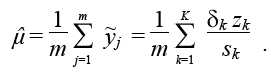C.1

Unbiasedness is established as follows. First, given that the strip is located uniformly at random, the probability that the strip will fall in a given column c of the grid that defines the population is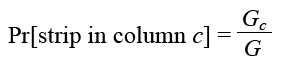C.2

where Gc is the total number of cells in column c (Sc  Gc = G). Second, conditional on the strip being located in column c the expected value of dk is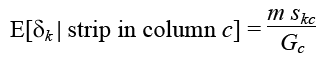C.3

where skc is the number of cells in network k and also in column c (Sc skc = sk). Strictly speaking, Eq. C.2 holds only for networks in the interior of the population or, for example, if the strip is reflected back upon itself when a population boundary is encountered (see Gregoire and Valentine 2008).  Combining results Eq. C.1 and Eq. C.2 yields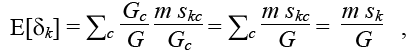and therefore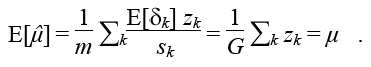If multiple strips are located independently at random then the dispersion among strip-wise estimates can be used to unbiasedly estimate the variance of Eq. 1 and to construct confidence intervals for µ.

LITERATURE CITED

Gregoire, T. G., and H. T. Valentine. 2008. Sampling strategies for natural resources and the environment. Taylor and Francis Group, Boca Raton, Florida, USA.

[Back to A021-026]